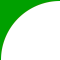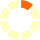# New York State Next Generation Learning Standards: 6.EE.9

## Use variables to represent two quantities in a real-world problem that change in relationship to one another.e.g., In a problem involving motion at constant speed, list and graph ordered pairs of distances and times.Given a verbal context and an equation, identify the dependent variable, in terms of the other quantity, thought of as the independent variable. Analyze the relationship between the dependent and independent variables using graphs and tables, and relate these to the equation.e.g., Given the equation d = 65t to represent the relationship between distance and time, identify t as the independent variable and d as the dependent variable.